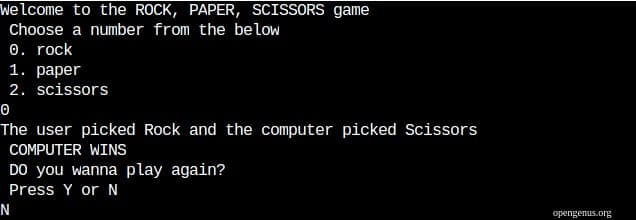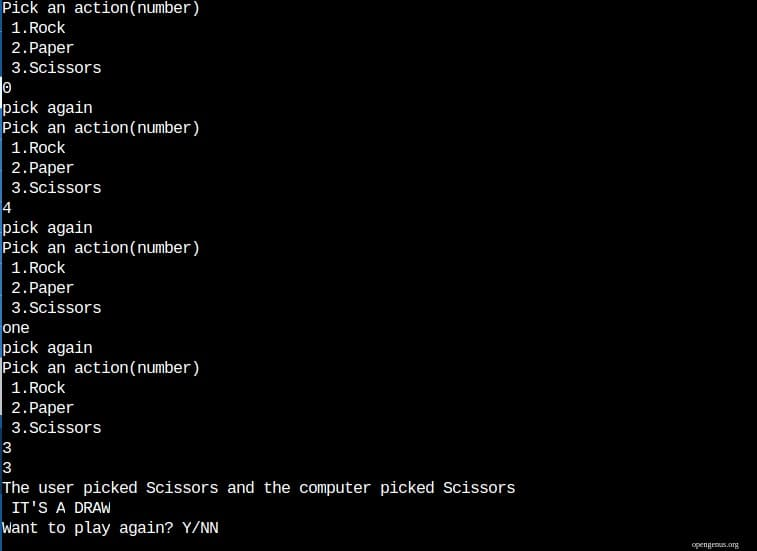×

Search anything:

# Rock Paper Scissors game in Python

#### PythonIn this article at OpenGenus, We will we looking to create a Rock Paper Scissors game in Python.

A game now-a-days needs GUI, but we will be focused on making a command line game (or) a console based game. This game will have the following structure:

• User Input
• Computer Input
• Compute
• Declare the winner

Let's start by thinking about how are we going to approach this. We need to jot down the points that are essential to make this game. Firstly, It's a game, so we would want to know if the user want's to play this game again. No one wants to loose a game to a computer, right?( gamers assemble). Secondly, we need to think about the type of input we want to take from the user, is it a number or a string. Thirdly, we need to think about the winning combinations or rules for this game to get to a clear winner or a loser. Fourthly, thinking about the cases where it would be a draw for both of them. Finally, declaring the winner.

For Rock Paper Scissors, the rules to determine a winner are:

1. If user1 picks Rock and user 2 picks Scissors, then user1 wins.
2. If user1 picks Paper and user2 picks Rock, then user1 wins.
3. If user1 picks Scissors and user2 picks Paper, then user1 wins.
4. In all the other cases user2 wins.
5. If both user1 and user2 pick a similar action then it will result in a draw.

If your question is why?, as per the game rules, paper is crushed by scissors, rock by paper and scissors by rock.

These quick ideas give us a structure in which we are gonna start building our game.

Variation-1

Here, we are going to use basic control flow statements like decision making statements and loop statements. The code in this variation will be:

``````from random import randint

print('Welcome to the ROCK, PAPER, SCISSORS game \n Choose a number from the below')

replayflag=True

while replayflag==True:
numbercheckflag=False

while numbercheckflag==False:

try:
user_no=int(input(" 0. rock \n 1. paper \n 2. scissors \n"))
if(user_no<0 or user_no>2):
print(' Pick again')
else:
numbercheckflag=True
except:
print('pick again')

options=['Rock', 'Paper', 'Scissors']

user_choice=options[user_no]

computer_no=randint(0,3)

print(f"The user picked {options[user_no]} and the computer picked {options[computer_no]}")

if ( user_no==computer_no):
print(" IT'S A DRAW ")

elif ((user_no==1 and computer_no==3) or (user_no==2 and computer_no==1) or (user_no==3 and computer_no==2)):
print(" USER WINS ")
else:
print(" COMPUTER WINS ")

rep_choice=input(' DO you wanna play again?\n Press Y or N\n')
replayflag= True if(rep_choice=='Y') else False

``````

Output:Explanation:

We are importing randint from random module to make computer choose random choices at will, if not it would be boring and not a game per se. We are presenting the user with the possible choices he has and taking their input as a number. This will help us in determing a winner later. Because we need to check whether a player wants to continue the game after an iteration we are using a flag. If the flag is set to true only then will it go for another iteration otherwise, it would stop. Now, we would be taking choice of the user, we are choosing a number. Because input taken from the user has to be a controlled input. If at all a user enters an input other than the one's we are going to process it should be handled. To do that, we are using another flag which will be set true only if the user input is a number and is choosing from [0,2] with 0 and 2 inclusive. To handle cases where if a user enters a string that would throw an exception so, we are using try and except block. Now that user input is done, we will take computer's input for that we are calling randint function with 0 and 2 as the arguments. This would be a number at random and return. We are using a list to get the required action that a particular number corresponds to although using numbers for decision making will be a lot easy to print information on screen it would help, remember a game should be responsive and give out information as and when it's necessary. We will use decision making control statements now to determine whether the choices of the user and the computer is leading to a draw or a win for the user or for the computer. The end would be to set the replay flag to 'True' or 'False' based on whether user wants to play or stop.

Variation-2

``````from enum import IntEnum
from random import randint

class Action(IntEnum):
Rock=1
Paper=2
Scissors=3

def get_action(number):

action=Action(number)
return action.name

replayflag=True
while replayflag==True:

user_no=int(input('Pick an action(number)\n 1.Rock \n 2.Paper \n 3.Scissors\n'))
try:
numcheck=False
while numcheck==False:
if(user_no<1 or user_no>3):
print('pick again')
else:
numcheck=True
except:
print('pick again')

computer_no=randint(1,3)

print(computer_no)

user_pick=get_action(user_no)
computer_pick=get_action(computer_no)

print(f"The user picked {user_pick} and the computer picked {computer_pick}")

if ( user_pick==computer_pick):
print(" IT'S A DRAW ")

elif ((user_pick==1 and computer_pick==3) or (user_pick==2 and computer_pick==1) or (user_pick==3 and computer_pick==2)):
print(" USER WINS ")
else:
print(" COMPUTER WINS ")

rep=input('Want to play again? Y/N\n')
replayflag= True if rep=='Y' else False

``````

Output:Explanation:

This variation of the code is similar in all aspects compared to the first except that we are just using another class called Action which is inheriting IntEnum. We are importing IntEnum from enum module for assigning the number to the particular action.

Closing Statements:

There are many ways to code the above problem, but we need to think about the code simplicity w.r.t modifying the rules and options if needed and making the process to code convenient.

#### Sathya Sai Ram P

CSE Student at Jawaharlal Nehru Technological University; Software Developer, Intern at OpenGenus.

Rock Paper Scissors game in Python